Main Content

# scatter

•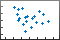## Syntax

``scatter(x,y)``
``scatter(x,y,sz)``
``scatter(x,y,sz,c)``
``scatter(___,'filled')``
``scatter(___,mkr)``
``scatter(___,Name,Value)``
``scatter(ax,___)``
``s = scatter(___)``

## Description

example

````scatter(x,y)` creates a scatter plot with circular markers at the locations specified by the vectors `x` and `y`. This type of graph is also known as a bubble plot. To plot one set of coordinates, specify `x` and `y` as vectors of equal length.To plot multiple sets of coordinates on the same set of axes, specify at least one of `x` or `y` as a matrix. ```

example

````scatter(x,y,sz)` specifies the circle sizes. To use the same size for all the circles, specify `sz` as a scalar. To plot each circle with a different size, specify `sz` as a vector or a matrix.```

example

````scatter(x,y,sz,c)` specifies the circle colors. You can specify one color for all the circles, or you can vary the color. For example, you can plot all red circles by specifying `c` as `'red'`.```

example

````scatter(___,'filled')` fills in the circles. Use the `'filled'` option with any of the input argument combinations in the previous syntaxes.```

example

````scatter(___,mkr)` specifies the marker type.```

example

````scatter(___,Name,Value)` modifies the scatter chart using one or more name-value pair arguments. For example, `'LineWidth',2` sets the marker outline width to 2 points.```

example

````scatter(ax,___)` plots into the axes specified by `ax` instead of into the current axes. The option `ax` can precede any of the input argument combinations in the previous syntaxes.```

example

````s = scatter(___)` returns the `Scatter` object or an array of `Scatter` objects. Use `s` to modify a scatter chart after creating it.```

## Examples

collapse all

Create `x` as 200 equally spaced values between 0 and $3\pi$. Create `y` as cosine values with random noise. Then, create a scatter plot.

```x = linspace(0,3*pi,200); y = cos(x) + rand(1,200); scatter(x,y)```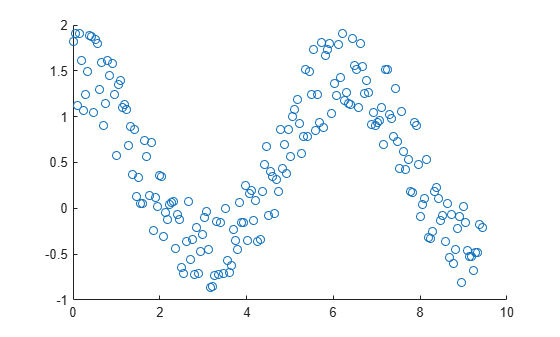Create a scatter plot using circles with different sizes. Specify the size in points squared

```x = linspace(0,3*pi,200); y = cos(x) + rand(1,200); sz = linspace(1,100,200); scatter(x,y,sz)```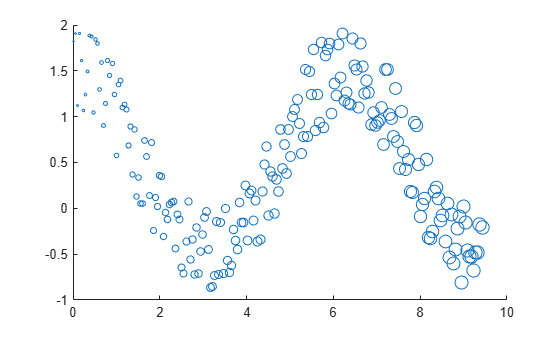Corresponding elements in `x`, `y`, and `sz` determine the location and size of each circle. To plot all circles with the equal area, specify `sz` as a numeric scalar.

Create a scatter plot and vary the circle color.

```x = linspace(0,3*pi,200); y = cos(x) + rand(1,200); c = linspace(1,10,length(x)); scatter(x,y,[],c)```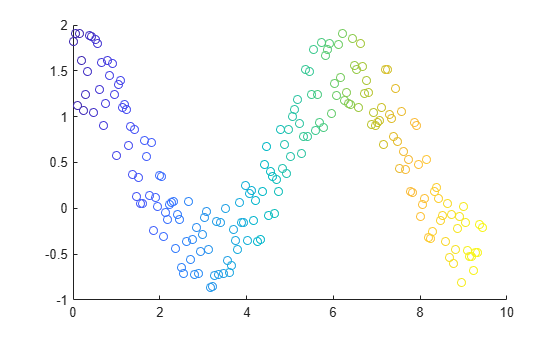Corresponding elements in `x`, `y`, and `c` determine the location and color of each circle. The `scatter` function maps the elements in `c` to colors in the current colormap.

Create a scatter plot and fill in the markers. `scatter` fills each marker using the color of the marker edge.

```x = linspace(0,3*pi,200); y = cos(x) + rand(1,200); sz = 25; c = linspace(1,10,length(x)); scatter(x,y,sz,c,'filled')```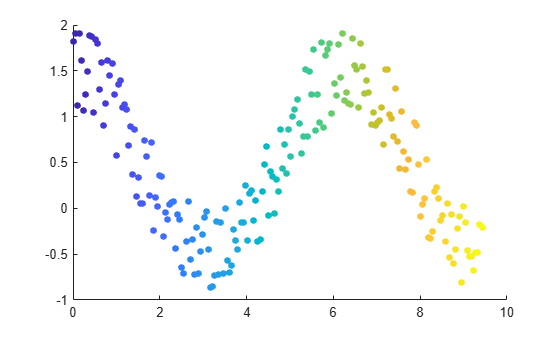Create vectors `x` and `y` as sine and cosine values with random noise. Then, create a scatter plot and use diamond markers with an area of 140 points squared.

```theta = linspace(0,2*pi,150); x = sin(theta) + 0.75*rand(1,150); y = cos(theta) + 0.75*rand(1,150); sz = 140; scatter(x,y,sz,'d')```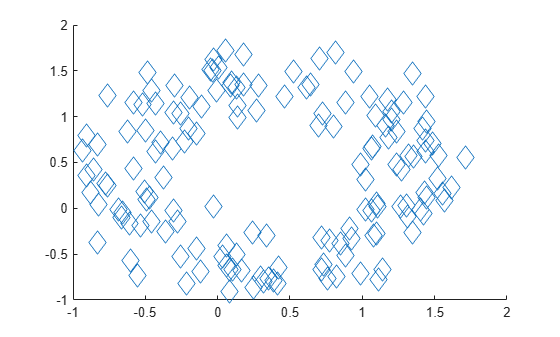Create vectors `x` and `y` as sine and cosine values with random noise. Create a scatter plot and set the marker edge color, marker face color, and line width.

```theta = linspace(0,2*pi,300); x = sin(theta) + 0.75*rand(1,300); y = cos(theta) + 0.75*rand(1,300); sz = 40; scatter(x,y,sz,'MarkerEdgeColor',[0 .5 .5],... 'MarkerFaceColor',[0 .7 .7],... 'LineWidth',1.5)```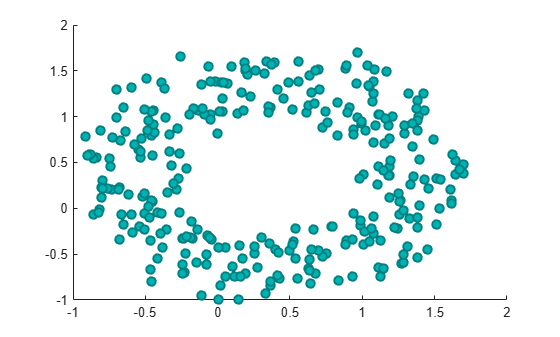You can vary the transparency of scattered points by setting the `AlphaData` property to a vector of different opacity values. To ensure the scatter plot uses the `AlphaData` values, set the `MarkerFaceAlpha` property to `'flat'`.

Create a set of normally distributed random numbers. Then create a scatter plot of the data with filled markers.

```x = randn(1000,1); y = randn(1000,1); s = scatter(x,y,'filled');```Set the opacity of each point according to its distance from zero.

```distfromzero = sqrt(x.^2 + y.^2); s.AlphaData = distfromzero; s.MarkerFaceAlpha = 'flat';```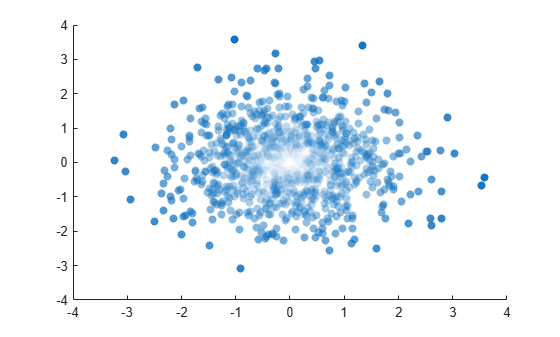Starting in R2019b, you can display a tiling of plots using the `tiledlayout` and `nexttile` functions. Call the `tiledlayout` function to create a 2-by-1 tiled chart layout. Call the `nexttile` function to create the axes objects `ax1` and `ax2`. Plot scattered data into each axes. In the bottom scatter plot, specify diamond filled diamond markers.

```x = linspace(0,3*pi,200); y = cos(x) + rand(1,200); tiledlayout(2,1) % Top plot ax1 = nexttile; scatter(ax1,x,y) % Bottom plot ax2 = nexttile; scatter(ax2,x,y,'filled','d')```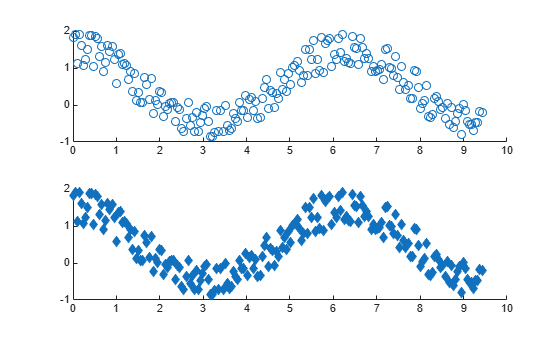Create a scatter plot and return the scatter series object, `s`.

```theta = linspace(0,1,500); x = exp(theta).*sin(100*theta); y = exp(theta).*cos(100*theta); s = scatter(x,y);```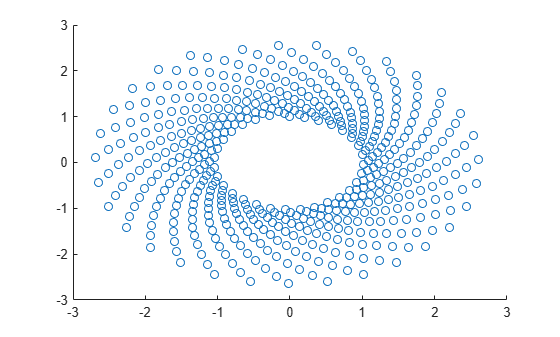Use `s` to query and set properties of the scatter series after it has been created. Set the line width to `0.6` point. Set the marker edge color to blue. Set the marker face color using an RGB triplet color.

```s.LineWidth = 0.6; s.MarkerEdgeColor = 'b'; s.MarkerFaceColor = [0 0.5 0.5];```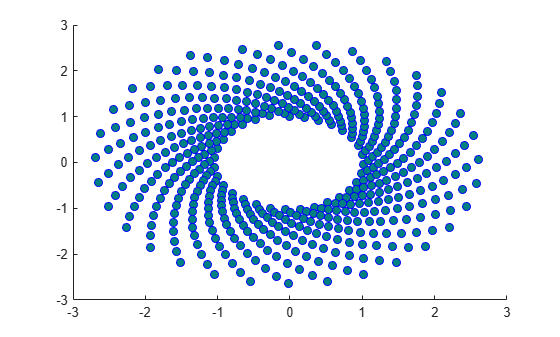## Input Arguments

collapse all

x-coordinates, specified as a scalar, vector, or matrix. The size and shape of `x` depends on the shape of your data. This table describes the most common situations.

Type of PlotHow to Specify Coordinates
Single point

Specify `x` and `y` as scalars. For example:

`scatter(1,2)`

One set of points

Specify `x` and `y` as any combination of row or column vectors of the same length. For example:

`scatter([1 2 3],[4; 5; 6])`

Multiple sets of points that are different colors

If all the sets share the same x- or y-coordinates, specify the shared coordinates as a vector and the other coordinates as a matrix. The length of the vector must match one of the dimensions of the matrix. For example:

`scatter([1 2 3],[4 5 6; 7 8 9])`
If the matrix is square, `scatter` plots a separate set of points for each column in the matrix.

Alternatively, specify `x` and `y` as matrices of equal size. In this case, `scatter` plots each column of `y` against the corresponding column of `x`. For example:

`scatter([1 3 5; 2 4 6],[10 25 45; 20 40 60])`

Data Types: `single` | `double` | `int8` | `int16` | `int32` | `int64` | `uint8` | `uint16` | `uint32` | `uint64` | `categorical` | `datetime` | `duration`

y-coordinates, specified as a scalar, vector, or matrix. The size and shape of `y` depends on the shape of your data. This table describes the most common situations.

Type of PlotHow to Specify Coordinates
Single point

Specify `x` and `y` as scalars. For example:

`scatter(1,2)`

One set of points

Specify `x` and `y` as any combination of row or column vectors of the same length. For example:

`scatter([1 2 3],[4; 5; 6])`

Multiple sets of points that are different colors

If all the sets share the same x- or y-coordinates, specify the shared coordinates as a vector and the other coordinates as a matrix. The length of the vector must match one of the dimensions of the matrix. For example:

`scatter([1 2 3],[4 5 6; 7 8 9])`
If the matrix is square, `scatter` plots a separate set of points for each column in the matrix.

Alternatively, specify `x` and `y` as matrices of equal size. In this case, `scatter` plots each column of `y` against the corresponding column of `x`. For example:

`scatter([1 3 5; 2 4 6],[10 25 45; 20 40 60])`

Data Types: `single` | `double` | `int8` | `int16` | `int32` | `int64` | `uint8` | `uint16` | `uint32` | `uint64` | `categorical` | `datetime` | `duration`

Marker size, specified as a numeric scalar, vector, matrix, or empty array (`[]`). The size controls the area of each marker in points squared. An empty array specifies the default size of 36 points. The way you specify the size depends on how you specify `x` and `y`, and how you want the plot to look. This table describes the most common situations.

Desired Marker Sizes`x` and `y` `sz`Example

Same size for all points

Any valid combination of vectors or matrices described for `x` and `y`.

Scalar

Specify `x` as a vector, `y` as a matrix, and `sz` as a scalar.

```x = [1 2 3 4]; y = [1 6; 3 8; 2 7; 4 9]; scatter(x,y,100)```

Different size for each point

Vectors of the same length

• A vector with the same length as `x` and `y`.

• A matrix with at least one dimension that matches the lengths of `x` and `y`. Specifying a matrix is useful for displaying multiple markers with different sizes at each (x,y) location.

Specify `x`, `y`, and `sz` as vectors.

```x = [1 2 3 4]; y = [1 3 2 4]; sz = [80 150 700 50]; scatter(x,y,sz)```

Specify `x` and `y` as vectors and `sz` as a matrix.

```x = [1 2 3 4]; y = [1 3 2 4]; sz = [80 30; 150 900; 50 2000; 200 350]; scatter(x,y,sz)```

Different size for each point

At least one of `x` or `y` is a matrix for plotting multiple data sets

• A vector with the same number of elements as there are points in each data set.

• A matrix that has the same size as the `x` or `y` matrix.

Specify `x` as a vector, `y` as a matrix, and `sz` as vector.

```x = [1 2 3 4]; y = [1 6; 3 8; 2 7; 4 9]; sz = [80 150 50 700]; scatter(x,y,sz)```

Specify `x` as a vector, `y` as a matrix, and `sz` as a matrix the same size as `y`.

```x = [1 2 3 4]; y = [1 6; 3 8; 2 7; 4 9]; sz = [80 30; 150 900; 50 2000; 200 350]; scatter(x,y,sz)```

Marker color, specified as a color name, RGB triplet, matrix of RGB triplets, or a vector of colormap indices.

• Color name — A color name such as `'red'`, or a short name such as `'r'`.

• RGB triplet — A three-element row vector whose elements specify the intensities of the red, green, and blue components of the color. The intensities must be in the range `[0,1]`; for example, ```[0.4 0.6 0.7]```. RGB triplets are useful for creating custom colors.

• Matrix of RGB triplets — A three-column matrix in which each row is an RGB triplet.

• Vector of colormap indices — A vector of numeric values that is the same length as the `x` and `y` vectors.

The way you specify the color depends on the desired color scheme and whether you are plotting one set of coordinates or multiple sets of coordinates. This table describes the most common situations.

Color SchemeHow to Specify the ColorExample

Use one color for all the points.

Specify a color name or a short name from the table below, or specify one RGB triplet.

Plot one set of points, and specify the color as `'red'`.

`scatter(1:4,[2 5 3 7],[],'red')`

Plot two sets of points, and specify the color as red using an RGB triplet.

`scatter(1:4,[2 5; 1 2; 8 4; 11 9],[],[1 0 0])`

Assign different colors to each point using a colormap.

Specify a row or column vector of numbers. The numbers index into the current colormap array. The smallest value maps to the first row in the colormap, and the largest value maps to the last row. The intermediate values map linearly to the intermediate rows.

If your plot has three points, specify a column vector to ensure the values are interpreted as colormap indices.

You can use this method only when `x`, `y`, and `sz` are all vectors.

Create a vector `c` that specifies four colormap indices. Plot four points using the colors from the current colormap. Then, change the colormap to `winter`.

```c = 1:4; scatter(1:4,[2 5 3 7],[],c) colormap(gca,'winter')```

Create a custom color for each point.

Specify an m-by-3 matrix of RGB triplets, where m is the number of points in the plot.

You can use this method only when `x`, `y`, and `sz` are all vectors.

Create a matrix `c` that specifies RGB triplets for green, red, gray, and purple. Then create a scatter plot of four points using those colors.

```c = [0 1 0; 1 0 0; 0.5 0.5 0.5; 0.6 0 1]; scatter(1:4,[2 5 3 7],[],c) ```

Create a different color for each data set.

Specify an n-by-3 matrix of RGB triplets, where n is the number of data sets.

You can use this method only when at least one of `x`, `y`, or `sz` is a matrix.

Create a matrix `c` that contains two RGB triplets. Then plot two data sets using those colors.

```c = [1 0 0; 0.6 0 1]; s = scatter(1:4,[2 5; 1 2; 8 4; 11 9],[],c) ```

#### Color Names and RGB Triplets for Common Colors

Color NameShort NameRGB TripletHexadecimal Color CodeAppearance
`'red'``'r'``[1 0 0]``'#FF0000'``'green'``'g'``[0 1 0]``'#00FF00'``'blue'``'b'``[0 0 1]``'#0000FF'``'cyan'` `'c'``[0 1 1]``'#00FFFF'``'magenta'``'m'``[1 0 1]``'#FF00FF'``'yellow'``'y'``[1 1 0]``'#FFFF00'``'black'``'k'``[0 0 0]``'#000000'``'white'``'w'``[1 1 1]``'#FFFFFF'`Here are the RGB triplets and hexadecimal color codes for the default colors MATLAB® uses in many types of plots.

RGB TripletHexadecimal Color CodeAppearance
`[0 0.4470 0.7410]``'#0072BD'``[0.8500 0.3250 0.0980]``'#D95319'``[0.9290 0.6940 0.1250]``'#EDB120'``[0.4940 0.1840 0.5560]``'#7E2F8E'``[0.4660 0.6740 0.1880]``'#77AC30'``[0.3010 0.7450 0.9330]``'#4DBEEE'``[0.6350 0.0780 0.1840]``'#A2142F'`Marker type, specified as one of the values listed in this table.

MarkerDescription
`'o'`Circle
`'+'`Plus sign
`'*'`Asterisk
`'.'`Point
`'x'`Cross
`'_'`Horizontal line
`'|'`Vertical line
`'s'`Square
`'d'`Diamond
`'^'`Upward-pointing triangle
`'v'`Downward-pointing triangle
`'>'`Right-pointing triangle
`'<'`Left-pointing triangle
`'p'`Pentagram
`'h'`Hexagram

Option to fill the interior of the markers, specified as `'filled'`. Use this option with markers that have a face, for example, `'o'` or `'square'`. Markers that do not have a face and contain only edges do not draw (`'+'`, `'*'`, `'.'`, and `'x'`).

The `'filled'` option sets the `MarkerFaceColor` property of the `Scatter` object to `'flat'` and the `MarkerEdgeColor` property to `'none'`, so the marker faces draw, but the edges do not.

Target axes, specified as an `Axes` object, a `PolarAxes` object, or a `GeographicAxes` object. If you do not specify the axes and if the current axes are Cartesian axes, then the `scatter` function uses the current axes. To plot into polar axes, specify the `PolarAxes` object as the first input argument or use the `polarscatter` function. To plot into geographic axes, specify the `GeographicAxes` object as the first input argument or use the `geoscatter` function.

### Name-Value Pair Arguments

Specify optional comma-separated pairs of `Name,Value` arguments. `Name` is the argument name and `Value` is the corresponding value. `Name` must appear inside quotes. You can specify several name and value pair arguments in any order as `Name1,Value1,...,NameN,ValueN`.

Example: `'MarkerFaceColor','red'` sets the marker face color to red.

The `Scatter` object properties listed here are only a subset. For a complete list, see Scatter Properties.

Marker outline color, specified `'flat'`, an RGB triplet, a hexadecimal color code, a color name, or a short name. The default value of `'flat'` uses colors from the `CData` property.

For a custom color, specify an RGB triplet or a hexadecimal color code.

• An RGB triplet is a three-element row vector whose elements specify the intensities of the red, green, and blue components of the color. The intensities must be in the range `[0,1]`; for example, ```[0.4 0.6 0.7]```.

• A hexadecimal color code is a character vector or a string scalar that starts with a hash symbol (`#`) followed by three or six hexadecimal digits, which can range from `0` to `F`. The values are not case sensitive. Thus, the color codes `'#FF8800'`, `'#ff8800'`, `'#F80'`, and `'#f80'` are equivalent.

Alternatively, you can specify some common colors by name. This table lists the named color options, the equivalent RGB triplets, and hexadecimal color codes.

Color NameShort NameRGB TripletHexadecimal Color CodeAppearance
`'red'``'r'``[1 0 0]``'#FF0000'``'green'``'g'``[0 1 0]``'#00FF00'``'blue'``'b'``[0 0 1]``'#0000FF'``'cyan'` `'c'``[0 1 1]``'#00FFFF'``'magenta'``'m'``[1 0 1]``'#FF00FF'``'yellow'``'y'``[1 1 0]``'#FFFF00'``'black'``'k'``[0 0 0]``'#000000'``'white'``'w'``[1 1 1]``'#FFFFFF'``'none'`Not applicableNot applicableNot applicableNo color

Here are the RGB triplets and hexadecimal color codes for the default colors MATLAB uses in many types of plots.

RGB TripletHexadecimal Color CodeAppearance
`[0 0.4470 0.7410]``'#0072BD'``[0.8500 0.3250 0.0980]``'#D95319'``[0.9290 0.6940 0.1250]``'#EDB120'``[0.4940 0.1840 0.5560]``'#7E2F8E'``[0.4660 0.6740 0.1880]``'#77AC30'``[0.3010 0.7450 0.9330]``'#4DBEEE'``[0.6350 0.0780 0.1840]``'#A2142F'`Example: `[0.5 0.5 0.5]`

Example: `'blue'`

Example: `'#D2F9A7'`

Marker fill color, specified as `'flat'`, `'auto'`, an RGB triplet, a hexadecimal color code, a color name, or a short name. The `'flat'` option uses the `CData` values. The `'auto'` option uses the same color as the `Color` property for the axes.

For a custom color, specify an RGB triplet or a hexadecimal color code.

• An RGB triplet is a three-element row vector whose elements specify the intensities of the red, green, and blue components of the color. The intensities must be in the range `[0,1]`; for example, ```[0.4 0.6 0.7]```.

• A hexadecimal color code is a character vector or a string scalar that starts with a hash symbol (`#`) followed by three or six hexadecimal digits, which can range from `0` to `F`. The values are not case sensitive. Thus, the color codes `'#FF8800'`, `'#ff8800'`, `'#F80'`, and `'#f80'` are equivalent.

Alternatively, you can specify some common colors by name. This table lists the named color options, the equivalent RGB triplets, and hexadecimal color codes.

Color NameShort NameRGB TripletHexadecimal Color CodeAppearance
`'red'``'r'``[1 0 0]``'#FF0000'``'green'``'g'``[0 1 0]``'#00FF00'``'blue'``'b'``[0 0 1]``'#0000FF'``'cyan'` `'c'``[0 1 1]``'#00FFFF'``'magenta'``'m'``[1 0 1]``'#FF00FF'``'yellow'``'y'``[1 1 0]``'#FFFF00'``'black'``'k'``[0 0 0]``'#000000'``'white'``'w'``[1 1 1]``'#FFFFFF'``'none'`Not applicableNot applicableNot applicableNo color

Here are the RGB triplets and hexadecimal color codes for the default colors MATLAB uses in many types of plots.

RGB TripletHexadecimal Color CodeAppearance
`[0 0.4470 0.7410]``'#0072BD'``[0.8500 0.3250 0.0980]``'#D95319'``[0.9290 0.6940 0.1250]``'#EDB120'``[0.4940 0.1840 0.5560]``'#7E2F8E'``[0.4660 0.6740 0.1880]``'#77AC30'``[0.3010 0.7450 0.9330]``'#4DBEEE'``[0.6350 0.0780 0.1840]``'#A2142F'`Example: `[0.3 0.2 0.1]`

Example: `'green'`

Example: `'#D2F9A7'`

Width of marker edge, specified as a positive value in point units.

Example: `0.75`

## Output Arguments

collapse all

`Scatter` object or an array of `Scatter` objects. Use `s` to modify properties of a scatter chart after creating it.

## See Also

### External Websites

Introduced before R2006a

Download ebook